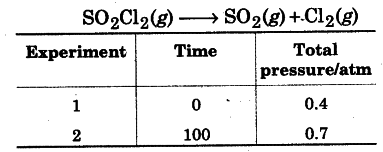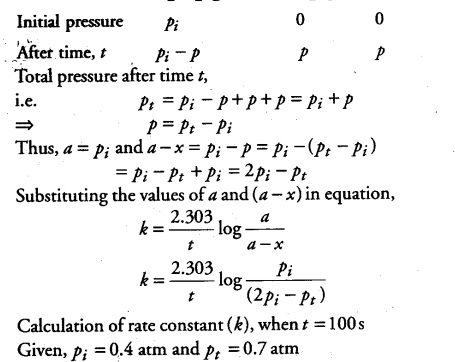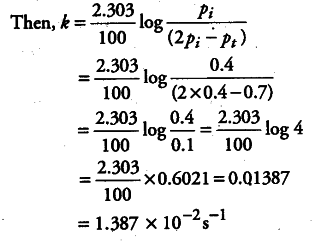# Calculate the rate constant

The following data were obtained during the first order thermal decomposition of So_{2}Cl_{2} at a constant volume:Calculate the rate constant.(Given, log 4 = 0.6021, log 2 = 0.3010)